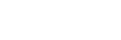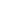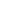# pytorch-custom-dataset-examples | Some custom dataset examples for PyTorch | Machine Learning library

by   utkuozbulak Python Version: Current License: MIT

by   utkuozbulak Python Version: Current License: MIT

## kandi X-RAY | pytorch-custom-dataset-examples Summary

pytorch-custom-dataset-examples is a Python library typically used in Artificial Intelligence, Machine Learning, Deep Learning, Pytorch applications. pytorch-custom-dataset-examples has no bugs, it has no vulnerabilities, it has a Permissive License and it has low support. However pytorch-custom-dataset-examples build file is not available. You can download it from GitHub.
Update after two years: It has been a long time since I have created this repository to guide people who are getting started with pytorch (like myself back then). However, over the course of years and various projects, the way I create my datasets changed many times. I included an additional bare bone dataset here to show what I am currently using. I would like to note that the reason why custom datasets are called custom is because you can shape it in anyway you desire. So, it is only natural that you (the reader) will develop your way of creating custom datasets after working on different projects. Examples presented in this project are not there as the ultimate way of creating them but instead, there to show the flexibility and the possiblity of pytorch datasets. I hope this repository is/was useful in your understanding of pytorch datasets. There are some official custom dataset examples on PyTorch repo like this but they still seemed a bit obscure to a beginner (like me, back then) so I had to spend some time understanding what exactly I needed to have a fully customized dataset. To save you the trouble of going through bajillions of pages, here, I decided to write down the basics of Pytorch datasets. The topics are as follows.
Support
Support
Quality
Quality
Security
Security
Reuse
ReuseSupport

• pytorch-custom-dataset-examples has a low active ecosystem.
• It has 687 star(s) with 105 fork(s). There are 20 watchers for this library.
• It had no major release in the last 12 months.
• There are 0 open issues and 6 have been closed. On average issues are closed in 71 days. There are no pull requests.
• It has a neutral sentiment in the developer community.
This Library - Support
Best in #Machine Learning
Average in #Machine Learning
This Library - Support
Best in #Machine Learning
Average in #Machine LearningQuality

• pytorch-custom-dataset-examples has 0 bugs and 0 code smells.
This Library - Quality
Best in #Machine Learning
Average in #Machine Learning
This Library - Quality
Best in #Machine Learning
Average in #Machine LearningSecurity

• pytorch-custom-dataset-examples has no vulnerabilities reported, and its dependent libraries have no vulnerabilities reported.
• pytorch-custom-dataset-examples code analysis shows 0 unresolved vulnerabilities.
• There are 0 security hotspots that need review.
This Library - Security
Best in #Machine Learning
Average in #Machine Learning
This Library - Security
Best in #Machine Learning
Average in #Machine LearningLicense

• Permissive licenses have the least restrictions, and you can use them in most projects.
Best in #Machine Learning
Average in #Machine Learning
Best in #Machine Learning
Average in #Machine LearningReuse

• pytorch-custom-dataset-examples releases are not available. You will need to build from source code and install.
• pytorch-custom-dataset-examples has no build file. You will be need to create the build yourself to build the component from source.
• Installation instructions are not available. Examples and code snippets are available.
• pytorch-custom-dataset-examples saves you 41 person hours of effort in developing the same functionality from scratch.
• It has 110 lines of code, 11 functions and 4 files.
• It has low code complexity. Code complexity directly impacts maintainability of the code.
This Library - Reuse
Best in #Machine Learning
Average in #Machine Learning
This Library - Reuse
Best in #Machine Learning
Average in #Machine Learning
Top functions reviewed by kandi - BETA

kandi has reviewed pytorch-custom-dataset-examples and discovered the below as its top functions. This is intended to give you an instant insight into pytorch-custom-dataset-examples implemented functionality, and help decide if they suit your requirements.

• Get a single image .
• Init the image list
• Forward convolution layer .
• The length of the data .

## pytorch-custom-dataset-examples Key Features

Some custom dataset examples for PyTorch

Custom Dataset FundamentalsCopyDownload
``````from torch.utils.data.dataset import Dataset

class MyCustomDataset(Dataset):
def __init__(self, ...):
# stuff

def __getitem__(self, index):
# stuff
return (img, label)

def __len__(self):
return count # of how many examples(images?) you have
``````

Using Torchvision TransformsCopyDownload
``````from torch.utils.data.dataset import Dataset
from torchvision import transforms

class MyCustomDataset(Dataset):
def __init__(self, ..., transforms=None):
# stuff
...
self.transforms = transforms

def __getitem__(self, index):
# stuff
...
data = # Some data read from a file or image
if self.transforms is not None:
data = self.transforms(data)
# If the transform variable is not empty
# then it applies the operations in the transforms with the order that it is created.
return (img, label)

def __len__(self):
return count # of how many data(images?) you have

if __name__ == '__main__':
# Define transforms (1)
transformations = transforms.Compose([transforms.CenterCrop(100), transforms.ToTensor()])
# Call the dataset
custom_dataset = MyCustomDataset(..., transformations)

``````

Another Way to Use Torchvision TransformsCopyDownload
``````from torch.utils.data.dataset import Dataset
from torchvision import transforms

class MyCustomDataset(Dataset):
def __init__(self, ...):
# stuff
...
# (2) One way to do it is define transforms individually
# When you define the transforms it calls __init__() of the transform
self.center_crop = transforms.CenterCrop(100)
self.to_tensor = transforms.ToTensor()

# (3) Or you can still compose them like
self.transformations = \
transforms.Compose([transforms.CenterCrop(100),
transforms.ToTensor()])

def __getitem__(self, index):
# stuff
...
data = # Some data read from a file or image

# When you call the transform for the second time it calls __call__() and applies the transform
data = self.center_crop(data)  # (2)
data = self.to_tensor(data)  # (2)

# Or you can call the composed version
data = self.transformations(data)  # (3)

# Note that you only need one of the implementations, (2) or (3)
return (img, label)

def __len__(self):
return count # of how many data(images?) you have

if __name__ == '__main__':
# Call the dataset
custom_dataset = MyCustomDataset(...)

``````

Incorporating PandasCopyDownload
``````class CustomDatasetFromImages(Dataset):
def __init__(self, csv_path):
"""
Args:
csv_path (string): path to csv file
img_path (string): path to the folder where images are
transform: pytorch transforms for transforms and tensor conversion
"""
# Transforms
self.to_tensor = transforms.ToTensor()
# First column contains the image paths
self.image_arr = np.asarray(self.data_info.iloc[:, 0])
# Second column is the labels
self.label_arr = np.asarray(self.data_info.iloc[:, 1])
# Third column is for an operation indicator
self.operation_arr = np.asarray(self.data_info.iloc[:, 2])
# Calculate len
self.data_len = len(self.data_info.index)

def __getitem__(self, index):
# Get image name from the pandas df
single_image_name = self.image_arr[index]
# Open image
img_as_img = Image.open(single_image_name)

# Check if there is an operation
some_operation = self.operation_arr[index]
# If there is an operation
if some_operation:
# Do some operation on image
# ...
# ...
pass
# Transform image to tensor
img_as_tensor = self.to_tensor(img_as_img)

# Get label(class) of the image based on the cropped pandas column
single_image_label = self.label_arr[index]

return (img_as_tensor, single_image_label)

def __len__(self):
return self.data_len

if __name__ == "__main__":
# Call dataset
custom_mnist_from_images =  \
CustomDatasetFromImages('../data/mnist_labels.csv')
``````

Incorporating Pandas with More LogicCopyDownload
``````class CustomDatasetFromCSV(Dataset):
def __init__(self, csv_path, height, width, transforms=None):
"""
Args:
csv_path (string): path to csv file
height (int): image height
width (int): image width
transform: pytorch transforms for transforms and tensor conversion
"""
self.labels = np.asarray(self.data.iloc[:, 0])
self.height = height
self.width = width
self.transforms = transform

def __getitem__(self, index):
single_image_label = self.labels[index]
# Read each 784 pixels and reshape the 1D array () to 2D array ([28,28])
img_as_np = np.asarray(self.data.iloc[index][1:]).reshape(28,28).astype('uint8')
# Convert image from numpy array to PIL image, mode 'L' is for grayscale
img_as_img = Image.fromarray(img_as_np)
img_as_img = img_as_img.convert('L')
# Transform image to tensor
if self.transforms is not None:
img_as_tensor = self.transforms(img_as_img)
# Return image and the label
return (img_as_tensor, single_image_label)

def __len__(self):
return len(self.data.index)

if __name__ == "__main__":
transformations = transforms.Compose([transforms.ToTensor()])
custom_mnist_from_csv = \
CustomDatasetFromCSV('../data/mnist_in_csv.csv', 28, 28, transformations)

``````

A Custom-custom-custom DatasetCopyDownload
``````1- Get the location of the image/data to read
3- Convert to numpy
4- Do some processing on numpy array (randomly)
5- Do some processing on numpy array (randomly)
6- Do some processing on numpy array (randomly)
...
15- Convert data to tensor
return location of data, name of data, data, and label
``````CopyDownload
``````...
if __name__ == "__main__":
# Define transforms
transformations = transforms.Compose([transforms.ToTensor()])
# Define custom dataset
custom_mnist_from_csv = \
CustomDatasetFromCSV('../data/mnist_in_csv.csv',
28, 28,
transformations)
batch_size=10,
shuffle=False)

# Feed the data to the model
``````

Multi-label, multi-class image classifier (ConvNet) with PyTorchCopyDownload
``````self.label_arr = np.asarray(self.data_info.iloc[:, 1:]) # columns 1 to N
``````
``````single_image_label = self.label_arr[index]
``````
-----------------------
``````self.label_arr = np.asarray(self.data_info.iloc[:, 1:]) # columns 1 to N
``````
``````single_image_label = self.label_arr[index]
``````

## Community Discussions

Trending Discussions on pytorch-custom-dataset-examples
###### Trending Discussions on pytorch-custom-dataset-examples

QUESTION

Multi-label, multi-class image classifier (ConvNet) with PyTorch

I am trying to implement an image classifier (CNN/ConvNet) with PyTorch where I want to read my labels from a csv-file. I have 4 different classes and an image may belong to more than one class.

I have read through the PyTorch Tutorial and this Stanford tutorial and this one, but none of them cover my specific case. I have managed to build a custom function of the `torch.utils.data.Dataset` class which works fine for reading the labels from a csv-file for a binary classifier only though.

This is the code for the `torch.utils.data.Dataset` class I have so far (slightly modified from the third tutorial linked above):

``````import torch
import torchvision.transforms as transforms
import torch.utils.data as data
from PIL import Image
import numpy as np
import pandas as pd

class MyCustomDataset(data.Dataset):
# __init__ function is where the initial logic happens like reading a csv,
# assigning transforms etc.
def __init__(self, csv_path):
# Transforms
self.random_crop = transforms.RandomCrop(800)
self.to_tensor = transforms.ToTensor()
# First column contains the image paths
self.image_arr = np.asarray(self.data_info.iloc[:, 0])
# Second column is the labels
self.label_arr = np.asarray(self.data_info.iloc[:, 1])
# Calculate len
self.data_len = len(self.data_info.index)

# __getitem__ function returns the data and labels. This function is
# called from dataloader like this
def __getitem__(self, index):
# Get image name from the pandas df
single_image_name = self.image_arr[index]
# Open image
img_as_img = Image.open(single_image_name)
img_cropped = self.random_crop(img_as_img)
img_as_tensor = self.to_tensor(img_cropped)

# Get label(class) of the image based on the cropped pandas column
single_image_label = self.label_arr[index]

return (img_as_tensor, single_image_label)

def __len__(self):
return self.data_len
``````

Specifically, I am trying to read my labels from a file with the following structure:And my specific problem is, that I can't figure out how to implement this into my `Dataset` class. I think I am missing the link between the (manual) assignment of the labels in the csv and how they are read by PyTorch, as I am rather new to the framework.
I'd appreciate any help on how to get this to work, or if there are actually examples covering this, a link would be highly appreciated as well!

Maybe I am missing something, but if you want to convert your columns `1..N` (`N = 4` here) into a label vector or shape `(N,)` (e.g. given your example data, `label(img1) = [0, 0, 0, 1]`, `label(img3) = [1, 0, 1, 0]`, ...), why not:

1. Read all the label columns into `self.label_arr`:

``````self.label_arr = np.asarray(self.data_info.iloc[:, 1:]) # columns 1 to N
``````
• Return accordingly the labels in `__getitem__()` (no change here):

• ``````single_image_label = self.label_arr[index]
``````

To train your classifier, you could then compute e.g. the cross-entropy between your `(N,)` predictions and the target labels.

Community Discussions, Code Snippets contain sources that include Stack Exchange Network

## Vulnerabilities

No vulnerabilities reported

## Install pytorch-custom-dataset-examples

You can use pytorch-custom-dataset-examples like any standard Python library. You will need to make sure that you have a development environment consisting of a Python distribution including header files, a compiler, pip, and git installed. Make sure that your pip, setuptools, and wheel are up to date. When using pip it is generally recommended to install packages in a virtual environment to avoid changes to the system.

## Support

For any new features, suggestions and bugs create an issue on GitHub. If you have any questions check and ask questions on community page Stack Overflow .

Explore Related Topics###### Find, review, and download reusable Libraries, Code Snippets, Cloud APIs from over 430 million Knowledge Items
Find more libraries
###### Reuse Solution Kits and Libraries Curated by Popular Use Cases

Save this library and start creating your kit

• © 2022 Open Weaver Inc.
• © 2022 Open Weaver Inc.